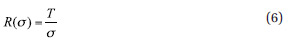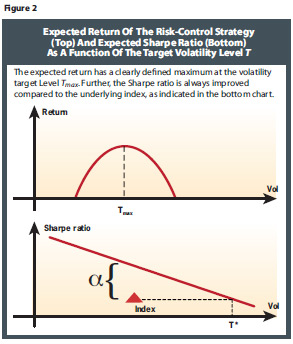Optimal Design Of Risk Control Strategy Indexes

August 17, 2012

Target-Risk/Target-Volatility Indexes
Another common type of strategy index that has been launched by many index providers is the so-called target volatility scheme, which aims to keep the volatility of the strategy index It at a predefined constant target level T, which is achieved by using the following response function in the concept of the strategy index (1):In essence, determining the equity investment according to (6) means that the volatility of the strategy index will be the equity weight times equity volatility T/?* ?=T, i.e., it is constant. At first glance, the advantage of the target volatility strategy is that it keeps the market risk of the investment strategy at a constant level. In particular, it reduces the size of the equity investment in turbulent markets, which are typically characterized by increasing levels of market volatility, and thereby protects investors from more serious losses when markets are falling. On the other hand, when market volatility is very low (which is typically the case in markets that are booming), the size of the equity investment is increased, possibly even above 100 percent to take advantage of leveraged returns.

At a second and more theoretical glance, the advantages of the target-volatility index scheme are even more pronounced: The mathematical analysis summarized in Appendix 3 online shows that the long-run Sharpe ratio of the target-volatility index is always greater than the Sharpe ratio of the underlying equity index, as long as the target volatility level T is chosen below a certain threshold T< T*, which is greater than the long-run average volatility of the equity index, i.e., T *>?.

Economically speaking, the reason for the improvement in the Sharpe ratio is the fact that the target volatility strategy is taking equity risk at the right point in time in the investment cycle: It takes more equity risk when the risk/reward profile of equity markets is very favorable (i.e., when volatility is low), and takes less equity risk and therefore a larger size of fixed-income investment when the risk/reward profile of equity markets is less favorable.

Further, as we show in the online appendixes, the improvement of the Sharpe ratio of the target volatility strategy compared with its underlying blue-chip index is proportional to the volatility of the underlying equity index. The economic explanation is again obvious: When volatility is itself very volatile, then there is more opportunity to improve the Sharpe ratio compared with a plain equity investment by investing cyclically, i.e., taking more equity risk in phases of low volatility and less equity risk in phases of high volatility. Therefore, the more cyclical volatility is, the greater the improvement of the Sharpe ratio becomes.

The mathematical results of the risk/return characteristics of target volatility indexes derived in the online appendixes are summarized in Figure 2.Figure 2 shows that the expected return of the target volatility index increases in the target volatility level up to a maximum Tmax. In other words, it does not make sense to choose a target volatility level above Tmax, because above this threshold expected returns decrease, while the risk increases.